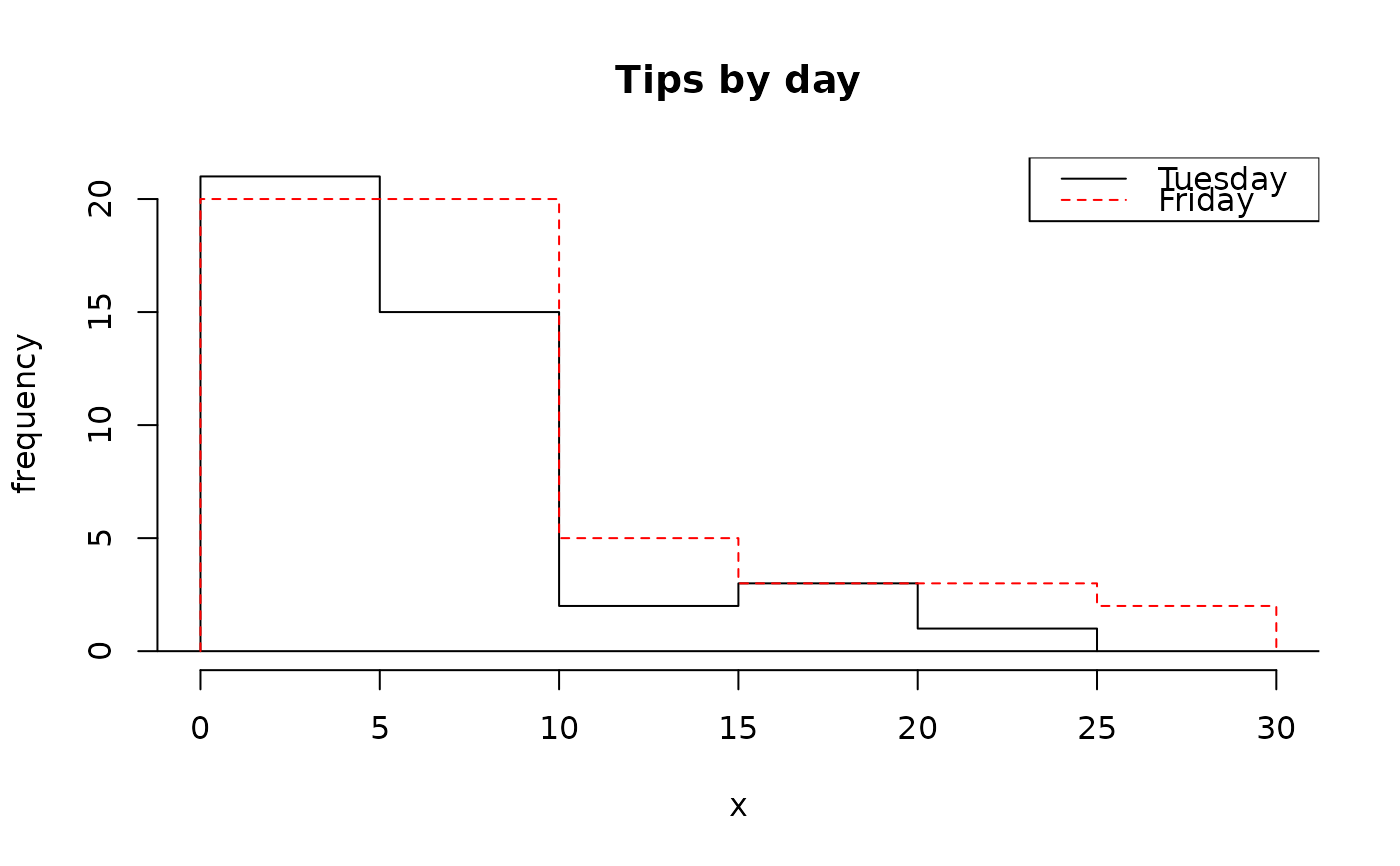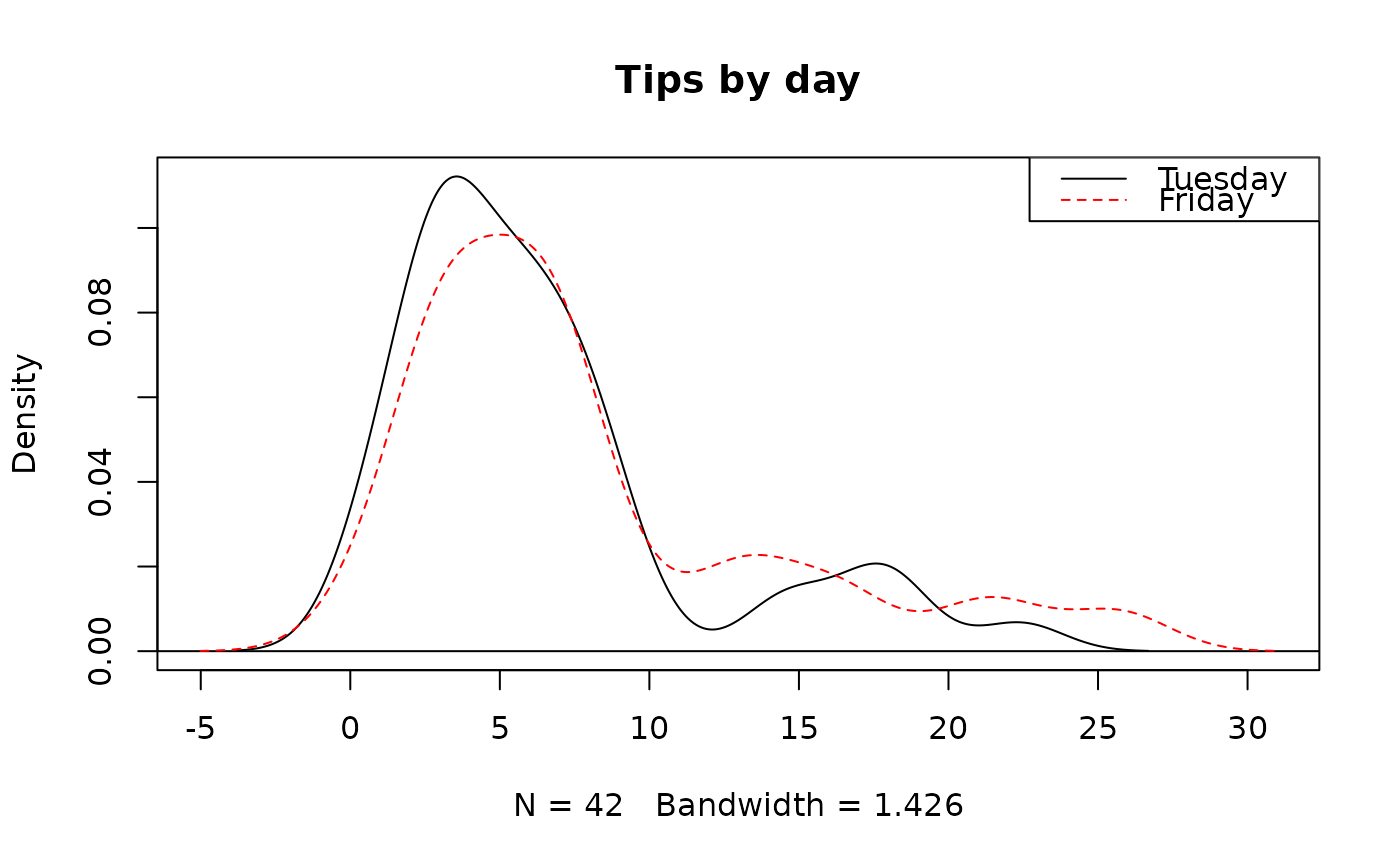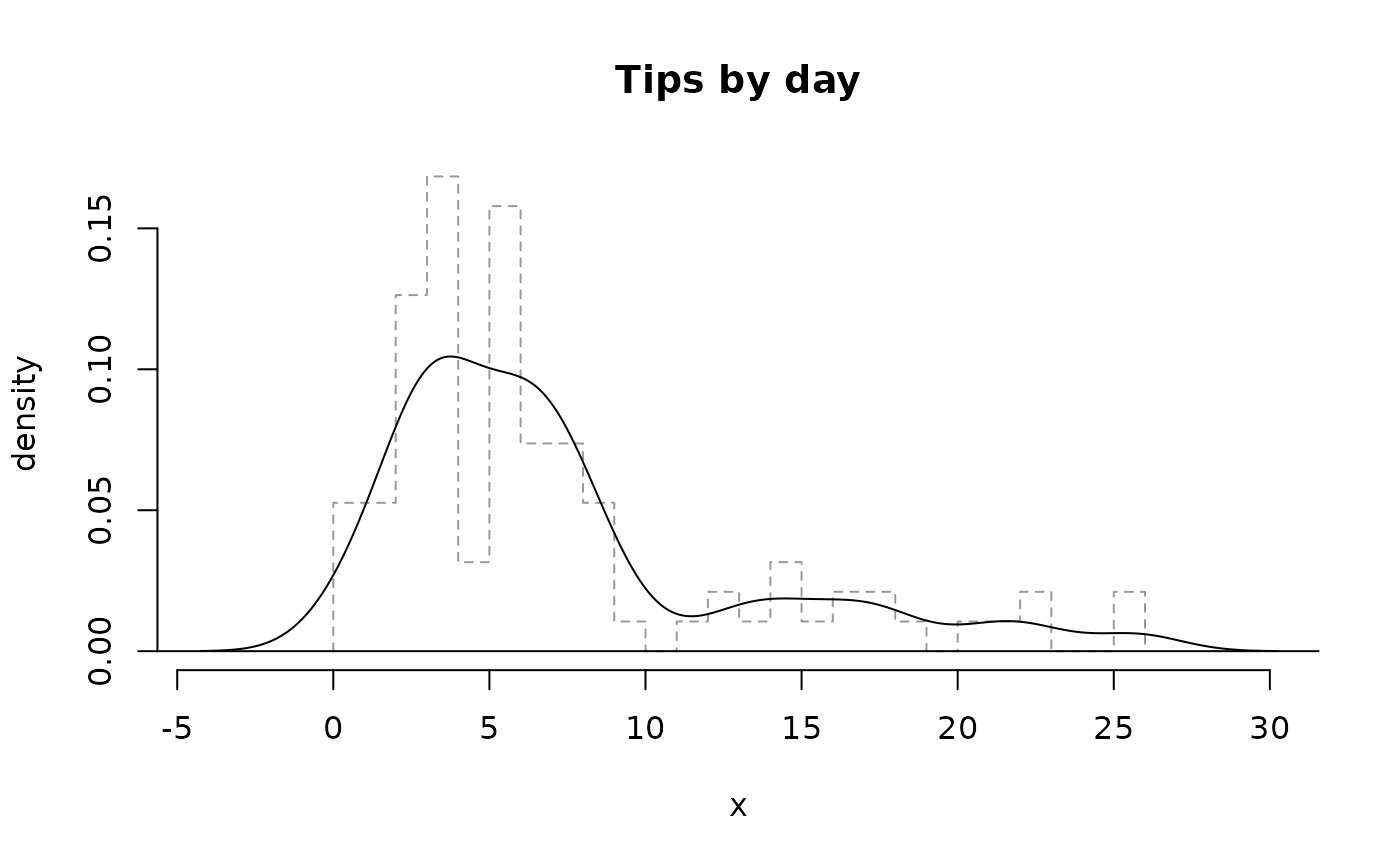Compute kernel density plots, written in the same structure as boxPlot. Histograms can be automatically added for teaching purposes.

## Usage

densityPlot(
x,
fact = NULL,
bw = "nrd0",
breaks = "Sturges",
lty = NULL,
lwd = 1,
col = c("black", "red", "blue"),
key = NULL,
kernel = c("gaussian", "epanechnikov", "rectangular", "triangular", "biweight",
"cosine", "optcosine"),
weights = NULL,
n = 512,
from,
to,
na.rm = FALSE,
xlim = NULL,
ylim = NULL,
main = "",
...
)

## Arguments

x

A numerical vector.

fact

A character or factor vector defining the grouping for data in x.

bw

Bandwidth. See density.

histo

Whether to plot a faded histogram ('faded') or hollow histogram ('hollow') in the background. By default, no histogram will be plotted.

breaks

The breaks argument for histPlot if histo is 'faded' or 'hollow'.

Character value of hexadecimal, e.g. '22' or '5D', describing the amount of fading inside the rectangles of the histogram if histo='faded'.

Character value of hexadecimal, e.g. '22' or '5D', describing the amount of fading of the rectangle borders of the histogram if histo is 'faded' or 'hollow'.

lty

Numerical vector describing the line type for the density curve(s). Each element corresponds to a different level of the argumentfact.

lwd

Numerical vector describing the line width for the density curve(s). Each element corresponds to a different level of the argumentfact.

col

Numerical vector describing the line color for the density curve(s). Each element corresponds to a different level of the argumentfact.

key

An argument to specify ordering of the factor levels.

If TRUE, the density curve is added to the plot.

Argument passed to density to adjust the bandwidth.

kernel

Argument passed to density to select the kernel used.

weights

Argument passed to density to weight observations.

n

Argument passed to density to specify the detail in the density estimate.

from

Argument passed to density specifying the lowest value to include in the density estimate.

to

Argument passed to density specifying the largest value to include in the density estimate.

na.rm

Argument passed to density specifying handling of NA values.

xlim

x-axis limits.

ylim

y-axis limits.

main

Title for the plot.

...

If add=FALSE, then additional arguments to plot.

histPlot, dotPlot, boxPlot

David Diez

## Examples


# hollow histograms
histPlot(tips$tip[tips$day == "Tuesday"],
hollow = TRUE, xlim = c(0, 30),
lty = 1, main = "Tips by day"
)
histPlot(tips$tip[tips$day == "Friday"],
hollow = TRUE, border = "red",
add = TRUE, main = "Tips by day"
)
legend("topright",
col = c("black", "red"),
lty = 1:2, legend = c("Tuesday", "Friday")
)# density plots
densityPlot(tips$tip, tips$day,
col = c("black", "red"), main = "Tips by day"
)
legend("topright",
col = c("black", "red"),
lty = 1:2, legend = c("Tuesday", "Friday")
)densityPlot(tips$tip, histo = "faded", breaks = 15, main = "Tips by day" )densityPlot(tips$tip,
histo = "hollow",
breaks = 30, fadingBorder = "66",
lty = 1, main = "Tips by day"
)